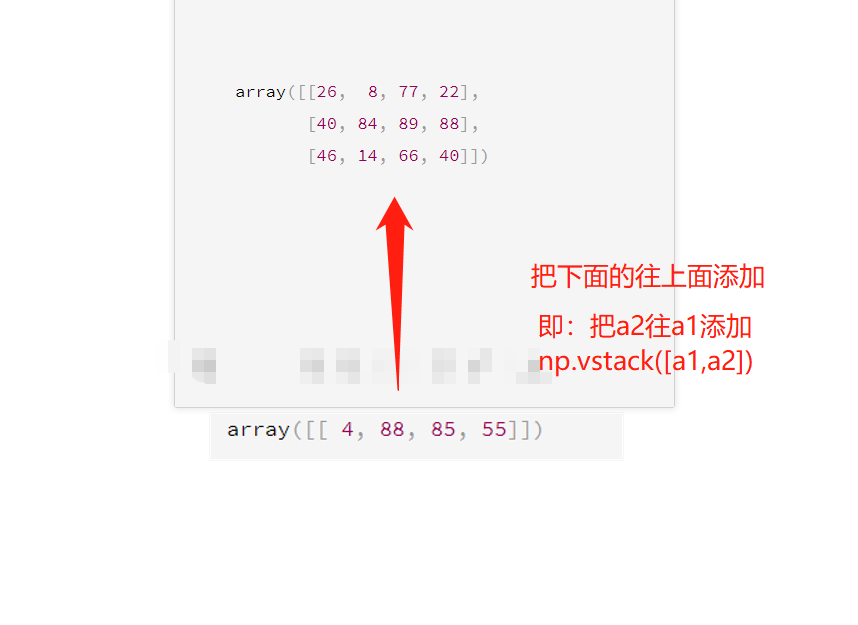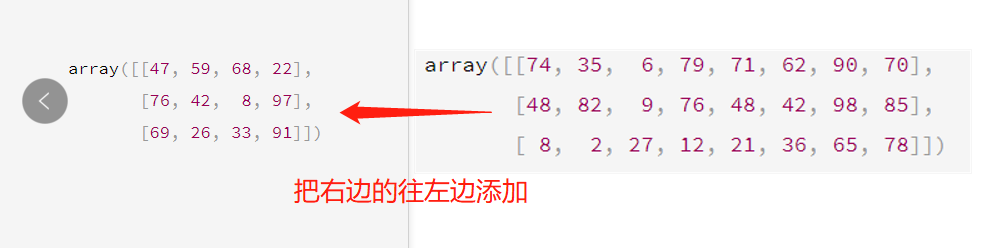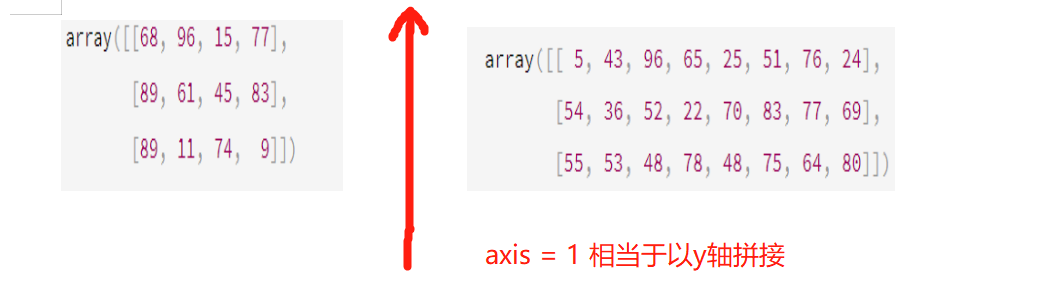• numpy数组拼接
千次阅读
2020-11-30 21:41:37

方法一:

Numpy中使用级联函数concatenate()来连接两个数组,可选参数为连接轴(连接维度) axis，axis默认为0，即默认在第0维上进行元素的连接。

• 级联函数使两数组指定维axis上的对应位置的元素相连接,若指定axis大小而时同维度上元素个数对应不相同时将会报错!
• 指定不同axis所得到的新数组形状不同

注：Numpy中维度从第0维开始

方法二:

使用堆栈函数stack()来连接数组，可选参数仍为连接轴axis，axis默认大小为0。

• 使用堆栈函数stack()连接数组与级联函数concatenate() 的区别在于stack()函数使两数组的元素按连接轴交替连接
• 指定不同的axis得到的数组形状相同

例子:

# -*- coding: utf-8 -*-
import numpy as np

arr1 = np.array([[[1, 2], [3, 4]],[[5, 6], [7, 8]]])

arr2 = np.array([[[9, 10], [11, 12]],[[13, 14], [15, 16]]])

"""级联拼接"""
arr = np.concatenate((arr1, arr2))               #默认在第0维上进行数组的连接

arr_1 = np.concatenate((arr1, arr2), axis=1)   #axis指定拼接的维度，axis=1表示在第1维上对数组进行拼接

arr_2 = np.concatenate((arr1, arr2), axis=2)   #axis指定拼接的维度，axis=2表示在第2维上对数组进行拼接

"""堆栈拼接"""
arr_3 = np.stack((arr1, arr2))                    #默认在第0维上进行数组的连接
arr_4 = np.stack((arr1, arr2),axis=1)             #在第1维上进行数组的连接
arr_5 = np.stack((arr1, arr2),axis=2)             #在第2维上进行数组的连接
arr_6 = np.stack((arr1, arr2),axis=3)             #在第3维上进行数组的连接

"""其他方法"""
arr_7 = np.hstack((arr1, arr2))                   #按行堆叠，相当于级联axis=1的方法

arr_8 = np.vstack((arr1, arr2))                   #按列堆叠，相当于级联axis=0的方法

arr_9 = np.dstack((arr1, arr2))                   #按深度堆叠，相当于级联axis=2的方法

print("原始数组:")
print(arr1)
print("数组类型:",arr1.shape)
print()
print(arr2)
print("数组类型:",arr2.shape)
print("--------------------------------")
print()

print("使用concatenate函数连接")
print()
print("默认在第0维上进行数组的连接")
print(arr)
print("数组类型:",arr.shape)
print("--------------------------------")
print()

print("--------------------------------")
print()
print("在第1维上进行数组的连接")
print(arr_1)
print("数组类型:",arr_1.shape)
print("--------------------------------")
print()

print("在第2维上进行数组的连接")
print(arr_2)
print("数组类型:",arr_2.shape)
print("--------------------------------")
print()

print("使用stack函数连接")
print()

print("默认在第0维上进行数组的连接")
print(arr_3)
print("数组类型:",arr_3.shape)
print("--------------------------------")
print()

print("在第1维上进行数组的连接")
print(arr_4)
print("数组类型:",arr_4.shape)
print("--------------------------------")
print()

print("在第2维上进行数组的连接")
print(arr_5)
print("数组类型:",arr_5.shape)
print("--------------------------------")
print()

print("在第3维上进行数组的连接")
print(arr_6)
print("数组类型:",arr_6.shape)
print("--------------------------------")
print()

print("按行堆叠")
print(arr_7)
print("数组类型:",arr_7.shape)
print("--------------------------------")
print()

print("按列堆叠")
print(arr_8)
print("数组类型:",arr_8.shape)
print("--------------------------------")
print()

print("按高(深)堆叠")
print(arr_9)
print("数组类型:",arr_9.shape)
print("--------------------------------")
print()

运行结果:


原始数组:
[[[1 2]
[3 4]]

[[5 6]
[7 8]]]
数组类型: (2, 2, 2)

[[[ 9 10]
[11 12]]

[[13 14]
[15 16]]]
数组类型: (2, 2, 2)
--------------------------------

使用concatenate函数连接

默认在第0维上进行数组的连接
[[[ 1  2]
[ 3  4]]

[[ 5  6]
[ 7  8]]

[[ 9 10]
[11 12]]

[[13 14]
[15 16]]]
数组类型: (4, 2, 2)
--------------------------------

--------------------------------

在第1维上进行数组的连接
[[[ 1  2]
[ 3  4]
[ 9 10]
[11 12]]

[[ 5  6]
[ 7  8]
[13 14]
[15 16]]]
数组类型: (2, 4, 2)
--------------------------------

在第2维上进行数组的连接
[[[ 1  2  9 10]
[ 3  4 11 12]]

[[ 5  6 13 14]
[ 7  8 15 16]]]
数组类型: (2, 2, 4)
--------------------------------

使用stack函数连接

默认在第0维上进行数组的连接
[[[[ 1  2]
[ 3  4]]

[[ 5  6]
[ 7  8]]]

[[[ 9 10]
[11 12]]

[[13 14]
[15 16]]]]
数组类型: (2, 2, 2, 2)
--------------------------------

在第1维上进行数组的连接
[[[[ 1  2]
[ 3  4]]

[[ 9 10]
[11 12]]]

[[[ 5  6]
[ 7  8]]

[[13 14]
[15 16]]]]
数组类型: (2, 2, 2, 2)
--------------------------------

在第2维上进行数组的连接
[[[[ 1  2]
[ 9 10]]

[[ 3  4]
[11 12]]]

[[[ 5  6]
[13 14]]

[[ 7  8]
[15 16]]]]
数组类型: (2, 2, 2, 2)
--------------------------------

在第3维上进行数组的连接
[[[[ 1  9]
[ 2 10]]

[[ 3 11]
[ 4 12]]]

[[[ 5 13]
[ 6 14]]

[[ 7 15]
[ 8 16]]]]
数组类型: (2, 2, 2, 2)
--------------------------------

按行堆叠
[[[ 1  2]
[ 3  4]
[ 9 10]
[11 12]]

[[ 5  6]
[ 7  8]
[13 14]
[15 16]]]
数组类型: (2, 4, 2)
--------------------------------

按列堆叠
[[[ 1  2]
[ 3  4]]

[[ 5  6]
[ 7  8]]

[[ 9 10]
[11 12]]

[[13 14]
[15 16]]]
数组类型: (4, 2, 2)
--------------------------------

按高(深)堆叠
[[[ 1  2  9 10]
[ 3  4 11 12]]

[[ 5  6 13 14]
[ 7  8 15 16]]]
数组类型: (2, 2, 4)
--------------------------------

Process finished with exit code 0python
更多相关内容
• NumPy数组是一个多维数组对象，称为ndarray。其由两部分组成： ·实际的数据 ·描述这些数据的元数据 大部分操作仅针对于元数据，而不改变底层实际的数据。 关于NumPy数组有几点必需了解的： ·NumPy数组的下标从0...
• 这篇文章主要介绍了numpy数组拼接简单示例，涉及对numpy数组的介绍，numpy数组的属性等内容，具有一定借鉴价值，需要的朋友可以参考下。NumPy数组是一个多维数组对象，称为ndarray。其由两部分组成：·实际的数据·...

这篇文章主要介绍了numpy数组拼接简单示例，涉及对numpy数组的介绍，numpy数组的属性等内容，具有一定借鉴价值，需要的朋友可以参考下。

NumPy数组是一个多维数组对象，称为ndarray。其由两部分组成：

·实际的数据

·描述这些数据的元数据

大部分操作仅针对于元数据，而不改变底层实际的数据。

关于NumPy数组有几点必需了解的：

·NumPy数组的下标从0开始。

·同一个NumPy数组中所有元素的类型必须是相同的。

NumPy数组属性

在详细介绍NumPy数组之前。先详细介绍下NumPy数组的基本属性。NumPy数组的维数称为秩（rank），一维数组的秩为1，二维数组的秩为2，以此类推。在NumPy中，每一个线性的数组称为是一个轴（axes），秩其实是描述轴的数量。比如说，二维数组相当于是两个一维数组，其中第一个一维数组中每个元素又是一个一维数组。所以一维数组就是NumPy中的轴（axes），第一个轴相当于是底层数组，第二个轴是底层数组里的数组。而轴的数量——秩，就是数组的维数。

NumPy的数组中比较重要ndarray对象属性有：

1.ndarray.ndim：数组的维数（即数组轴的个数），等于秩。最常见的为二维数组（矩阵）。

2.ndarray.shape：数组的维度。为一个表示数组在每个维度上大小的整数元组。例如二维数组中，表示数组的“行数”和“列数”。ndarray.shape返回一个元组，这个元组的长度就是维度的数目，即ndim属性。

3.ndarray.size：数组元素的总个数，等于shape属性中元组元素的乘积。

4.ndarray.dtype：表示数组中元素类型的对象，可使用标准的Python类型创建或指定dtype。另外也可使用前一篇文章中介绍的NumPy提供的数据类型。

5.ndarray.itemsize：数组中每个元素的字节大小。例如，一个元素类型为float64的数组itemsiz属性值为8(float64占用64个bits，每个字节长度为8，所以64/8，占用8个字节），又如，一个元素类型为complex32的数组item属性为4（32/8）。

6.ndarray.data：包含实际数组元素的缓冲区，由于一般通过数组的索引获取元素，所以通常不需要使用这个属性。

数组拼接方法一

思路：首先将数组转成列表，然后利用列表的拼接函数append()、extend()等进行拼接处理，最后将列表转成数组。

示例1：

>>> import numpy as np

>>> a=np.array([1,2,5])

>>> b=np.array([10,12,15])

>>> a_list=list(a)

>>> b_list=list(b)

>>> a_list.extend(b_list)

>>> a_list

[1, 2, 5, 10, 12, 15]

>>> a=np.array(a_list)

>>> a

array([ 1, 2, 5, 10, 12, 15])

该方法只适用于简单的一维数组拼接，由于转换过程很耗时间，对于大量数据的拼接一般不建议使用。

数组拼接方法二

思路：numpy提供了numpy.append(arr,values,axis=None)函数。对于参数规定，要么一个数组和一个数值；要么两个数组，不能三个及以上数组直接append拼接。append函数返回的始终是一个一维数组。

示例2：

>>> a=np.arange(5)

>>> a

array([0, 1, 2, 3, 4])

>>> np.append(a,10)

array([ 0, 1, 2, 3, 4, 10])

>>> a

array([0, 1, 2, 3, 4])

>>> b=np.array([11,22,33])

>>> b

array([11, 22, 33])

>>> np.append(a,b)

array([ 0, 1, 2, 3, 4, 11, 22, 33])

>>> a

array([[1, 2, 3],

[4, 5, 6]])

>>> b=np.array([[7,8,9],[10,11,12]])

>>> b

array([[ 7, 8, 9],

[10, 11, 12]])

>>> np.append(a,b)

array([ 1, 2, 3, 4, 5, 6, 7, 8, 9, 10, 11, 12])

numpy的数组没有动态改变大小的功能，numpy.append()函数每次都会重新分配整个数组，并把原来的数组复制到新数组中。

数组拼接方法三

思路：numpy提供了numpy.concatenate((a1,a2,...),axis=0)函数。能够一次完成多个数组的拼接。其中a1,a2,...是数组类型的参数

示例3：

>>> a=np.array([1,2,3])

>>> b=np.array([11,22,33])

>>> c=np.array([44,55,66])

>>> np.concatenate((a,b,c),axis=0) # 默认情况下，axis=0可以不写

array([ 1, 2, 3, 11, 22, 33, 44, 55, 66]) #对于一维数组拼接，axis的值不影响最后的结果

>>> a=np.array([[1,2,3],[4,5,6]])

>>> b=np.array([[11,21,31],[7,8,9]])

>>> np.concatenate((a,b),axis=0)

array([[ 1, 2, 3],

[ 4, 5, 6],

[11, 21, 31],

[ 7, 8, 9]])

>>> np.concatenate((a,b),axis=1) #axis=1表示对应行的数组进行拼接

array([[ 1, 2, 3, 11, 21, 31],

[ 4, 5, 6, 7, 8, 9]])

对numpy.append()和numpy.concatenate()两个函数的运行时间进行比较

示例4：

>>> from time import clock as now

>>> a=np.arange(9999)

>>> b=np.arange(9999)

>>> time1=now()

>>> c=np.append(a,b)

>>> time2=now()

>>> print time2-time1

28.2316728446

>>> a=np.arange(9999)

>>> b=np.arange(9999)

>>> time1=now()

>>> c=np.concatenate((a,b),axis=0)

>>> time2=now()

>>> print time2-time1

20.3934997107

可知，concatenate()效率更高，适合大规模的数据拼接

总结

以上就是本文关于numpy数组拼接简单示例的全部内容，希望对大家有所帮助。感兴趣的朋友可以继续参阅本站：

相关推荐：

以上就是numpy数组拼接简单示例_python的详细内容，更多请关注php中文网其它相关文章！本文原创发布php中文网，转载请注明出处，感谢您的尊重！

展开全文python矩阵拼接
• 1.数组拼接 1.行的拼接 vstack方法 import numpy as np a1 = np.random.randint(1,100,size = (3,4)) a2 = np.random.randint(1,100,size = (1,4)) a1 array([[26, 8, 77, 22], [40, 84, 89, 88], [46, 14, 66...

# vstack方法

import numpy as np
a1 = np.random.randint(1,100,size = (3,4))
a2 = np.random.randint(1,100,size = (1,4))
a1
array([[26,  8, 77, 22],
[40, 84, 89, 88],
[46, 14, 66, 40]])
a2
array([[ 4, 88, 85, 55]])

• 将俩个行不相同列相同的数组进行拼接
• 把 a2往 a1上加 np.vstack([a1,a2])

从下往上加a3 = np.vstack([a1,a2])
a3
array([[26,  8, 77, 22],
[40, 84, 89, 88],
[46, 14, 66, 40],
[ 4, 88, 85, 55]])


# hstack方法

import numpy as np
a1 = np.random.randint(1,100,size = (3,4))
a2 = np.random.randint(1,100,size = (3,7))
a1
array([[47, 59, 68, 22],
[76, 42,  8, 97],
[69, 26, 33, 91]])
a2
array([[74, 35,  6, 79, 71, 62, 90, 70],
[48, 82,  9, 76, 48, 42, 98, 85],
[ 8,  2, 27, 12, 21, 36, 65, 78]])

• 将俩个行相同列不相同的数组进行拼接
• 就是 a2从右边往 a1的左边加

从右往左加a3 = np.hstack([a1,a2])
a3
array([[47, 59, 68, 22, 74, 35,  6, 79, 71, 62, 90, 70],
[76, 42,  8, 97, 48, 82,  9, 76, 48, 42, 98, 85],
[69, 26, 33, 91,  8,  2, 27, 12, 21, 36, 65, 78]])


# concatenate方法a1
array([[68, 96, 15, 77],
[89, 61, 45, 83],
[89, 11, 74,  9]])
a2
array([[ 5, 43, 96, 65, 25, 51, 76, 24],
[54, 36, 52, 22, 70, 83, 77, 69],
[55, 53, 48, 78, 48, 75, 64, 80]])

np.concatenate((a1,a2),axis = 1)
array([[68, 96, 15, 77,  5, 43, 96, 65, 25, 51, 76, 24],
[89, 61, 45, 83, 54, 36, 52, 22, 70, 83, 77, 69],
[89, 11, 74,  9, 55, 53, 48, 78, 48, 75, 64, 80]])



# a1.T

a1
array([[68, 96, 15, 77],
[89, 61, 45, 83],
[89, 11, 74,  9]])

a1.T
array([[68, 89, 89],
[96, 61, 11],
[15, 45, 74],
[77, 83,  9]])


# 2.数组的分割

a = np.arange(16).reshape((4,4))
a
array([[ 0,  1,  2,  3],
[ 4,  5,  6,  7],
[ 8,  9, 10, 11],
[12, 13, 14, 15]])


# array_spli

np.array_split(a,2,axis=0) axis = 0以x轴进行分割

np.vsplit(a,2)

[array([[0, 1, 2, 3],
[4, 5, 6, 7]]),
array([[ 8,  9, 10, 11],
[12, 13, 14, 15]])]


# array_split

np.array_split(a,2,axis=1)  以y轴分割axis=1

np.hsplit(a,2)

[array([[ 0,  1],
[ 4,  5],
[ 8,  9],
[12, 13]]),
array([[ 2,  3],
[ 6,  7],
[10, 11],
[14, 15]])]

展开全文python 机器学习
• 数组拼接方法一 思路：首先将数组转成列表，然后利用列表的拼接函数append()、extend()等进行拼接处理，最后将列表转成数组。 示例1： import numpy as np a=np.array([1,2,5]) b=np.array([10,12,15]) a_list=...

方法一：数组拼接

思路：首先将数组转成列表，然后利用列表的拼接函数append()、extend()等进行拼接处理，最后将列表转成数组。

示例1：

>>> import numpy as np
>>> a=np.array([1,2,5])
>>> b=np.array([10,12,15])
>>> a_list=list(a)
>>> b_list=list(b)
>>> a_list.extend(b_list)
>>> a_list
[1, 2, 5, 10, 12, 15]
>>> a=np.array(a_list)
>>> a
array([ 1,  2,  5, 10, 12, 15])


该方法只适用于简单的一维数组拼接，由于转换过程很耗时间，对于大量数据的拼接一般不建议使用。

方法二：数组拼接

思路：numpy提供了numpy.append(arr, values, axis=None)函数。对于参数规定，要么一个数组和一个数值；要么两个数组，不能三个及以上数组直接append拼接。

示例2：

>>> a=np.arange(5)
>>> a
array([0, 1, 2, 3, 4])
>>> np.append(a,10)
array([ 0,  1,  2,  3,  4, 10])
>>> a
array([0, 1, 2, 3, 4])

>>> b=np.array([11,22,33])
>>> b
array([11, 22, 33])
>>> np.append(a,b)
array([ 0,  1,  2,  3,  4, 11, 22, 33])

>>> a
array([[1, 2, 3],
[4, 5, 6]])
>>> b=np.array([[7,8,9],[10,11,12]])
>>> b
array([[ 7,  8,  9],
[10, 11, 12]])
>>> np.append(a,b)
array([ 1,  2,  3,  4,  5,  6,  7,  8,  9, 10, 11, 12])


numpy的数组没有动态改变大小的功能，numpy.append()函数每次都会重新分配整个数组，并把原来的数组复制到新数组中。

方法三：数组拼接

思路：numpy提供了numpy.concatenate((a1,a2,…), axis=0)函数。能够一次完成多个数组的拼接。其中a1,a2,…是数组类型的参数

示例3：

>>> a=np.array([1,2,3])
>>> b=np.array([11,22,33])
>>> c=np.array([44,55,66])
>>> np.concatenate((a,b,c),axis=0)  # 默认情况下，axis=0可以不写
array([ 1,  2,  3, 11, 22, 33, 44, 55, 66]) #对于一维数组拼接，axis的值不影响最后的结果

>>> a=np.array([[1,2,3],[4,5,6]])
>>> b=np.array([[11,21,31],[7,8,9]])
>>> np.concatenate((a,b),axis=0)
array([[ 1,  2,  3],
[ 4,  5,  6],
[11, 21, 31],
[ 7,  8,  9]])

>>> np.concatenate((a,b),axis=1)  #axis=1表示对应行的数组进行拼接
array([[ 1,  2,  3, 11, 21, 31],
[ 4,  5,  6,  7,  8,  9]])


对numpy.append()和numpy.concatenate()两个函数的运行时间进行比较

示例4：

>>> from time import clock as now
>>> a=np.arange(9999)
>>> b=np.arange(9999)
>>> time1=now()
>>> c=np.append(a,b)
>>> time2=now()
>>> print time2-time1
28.2316728446
>>> a=np.arange(9999)
>>> b=np.arange(9999)
>>> time1=now()
>>> c=np.concatenate((a,b),axis=0)
>>> time2=now()
>>> print time2-time1
20.3934997107


可知，concatenate()效率更高，适合大规模的数据拼接

展开全文• 获取行或列【2×03】布尔索引【2×04】花式索引【3×00】NumPy 切片【4×00】NumPy 数组运算以及广播原则【7×00】数组拼接与元素的添加【7×01】将数组转换成列表，拼接完成再转换成数组【7×02】numpy.append()...
• 数组拼接主要函数有： np.hstack((a, b)) (水平拼接) np.vstack((a, b)) (竖直拼接) np.concatenate((a, b),axis=1) (水平拼接) np.concatenate((a, b),axis=0) (竖直拼接) import numpy as np a = np.arange...
• 数组或者张量的拼接和合成： 数组a,b: np.vstack((a, b)) v 表示vertical 垂直，也就是竖着拼接 ,行增加 np.hstack((a, b)) h表示Horizontal 横向，列增加 # 将POI和build特征合并 node_feat = np.hstack((Build_...
• 1.The Basics1.1 numpy 数组基础NumPy’s array class is called ndarray.ndarray.ndimthe number of axes (dimensions) of the array. In the Python world, the number of dimensions is referred to as rank....python数组拆分
• 数组拼接方法一 思路：首先将数组转成列表，然后利用列表的拼接函数append()、extend()等进行拼接处理，最后将列表转成数组。 示例1： &gt;&gt;&gt; import numpy as np &gt;&gt;&gt; a=...
• ## numpy数组拼接方法

千次阅读 2018-06-06 16:58:42
方法一： 转为list，使用“+”, append()或者extend() 可做简单的拼接。不过要注意一些小问题，如下图： 方法二：使用numpy提供的函数-append(arr, values, axis=None) 不能对三个或者三个以上数组...python
• 本文转载自：https://www.cnblogs.com/huangshiyu13/p/6672828.html 作者：huangshiyu13 转载请注明该声明。...============改变数组的维度==================已知reshape函数可以有一维数组形成多维数组ra...python 数组
• 拼接一个数组和一个数值 拼接两个数组 concatenate()函数 append()、extend()等拼接列表并将其转成数组，所以要先将数组转成列表。 extend()函数 只适用于简单的一维数组，对于大量数据的拼接一般不建议使用。...
• python下将两个三维数组拼接为一个数组的方式： import numpy as np l1 = np.zeros((7,8,10), np.float32) l2 = np.ones((7,8,10), np.float32) L1 = np.vstack((l1,l2))#纵向拼接（shape方向） L2 = np.hstack...
• 假设我们有一个3×2 numpy数组： x = array(([[1,2], [3, 4], [5,6]])) 现在需要把它与一个一维数组： y = array(([7, 8,9])) 通过将其添加到行的末尾，连接为一个3×3 numpy数组，如下所示： array([[1,2,7], ...
• 1.数组拼接方法一 思路：首先将数组转成列表，然后利用列表的拼接函数append()、extend()等进行拼接处理，最后将列表转成数组。 示例1： >>> import numpy as np >>> a=np.array([1,2,5]) &...python
• 我就写一下我遇到的，更多具体的请看Python之Numpy数组拼接，组合，连接 >>> aarray(［0, 1, 2],[3, 4, 5],[6, 7, 8］)>>> b = a*2>>> barray(［ 0, 2, 4],[ 6, 8, 10],[12, 14, 16］...
• append()、extend()等拼接列表并将其转成数组，所以要先将数组转成列表。 1.extend()函数 只适用于简单的一维数组，对于大量数据的拼接一般不建议使用。 import numpy as np a=np.array([1,2,5]) b=np.array([10,12,...python
• 方法一 使用np.c_[]和np.r_[]分别添加行和列 方法二 np.concatenate((a1, a2, …), axis=0) 方法三 使用np.insert(a, 0, values=b, axis=1) ps：只能插入行向量？...import numpy as np a = np.ar......